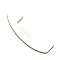# Credentialed Math Tutors

Mathematics is one of the most common subjects that students of all ages struggle with. At REACH Pro Tutoring, we are very experienced in helping students gain a stronger understanding in all levels of math.

## Experienced and Credentialed Math Tutors

Mathematics is one of the most common subjects that students of all ages struggle with. At REACH Pro Tutoring, we are very experienced in helping students gain a stronger understanding in all levels of math.
Attempting to introduce supplemental curriculum to a struggling student causes confusion and further frustration. To help avoid these issues, we employ private math tutors that are experienced in teaching the current common core standards to ensure our instruction is aligned with your child’s school curriculum. Students in middle and high school will be partnered with specialized single subject math tutors, while elementary students will have tutors with multiple subject credentials.

Our credentialed math tutors teach math because they love it, and their skills and enthusiasm help students begin to enjoy math class again. We work to not only improve student’s grades, but also their self-esteem and general attitude towards school.

#### Basic math

Basic math includes most math topics covered in elementary school, and vary depending on the age of your student. While the youngest students may just be learning how to count, other students may struggle with multiplication tables, long division, and word problems. Regardless of the topic, our private math tutors help students understand how to approach these math fundamentals through patient practice.

#### Pre-Algebra

In pre-algebra, graphing a system of linear equations is one of the most common areas that students struggle with. This level of math introduces students to integers, decimals, and negative numbers, so there are a lot of changes from natural number arithmetic to contend with. From the basic equations to factoring and everything in between, our math tutors will help your student master all the instrumental pre-algebra skills to prepare them for more advanced topics.

#### Algebra

Elementary algebra is considered to be essential for any professional study of mathematics, science or engineering. In the basic sense, algebra is the branch of mathematics that uses letters in place of unknown numbers. Algebra dives into the concepts of linear equations and graphing, and includes more challenges like exponents, logarithms, and functions.

#### Geometry

Geometry is a type of mathematics that is concerned with the properties of shapes, space, size, and relative position of objects. Students begin with plane geometry concepts like points, lines, and circles before moving to three-dimensional objects, volume, and more. Geometry is vital to understanding the concepts of measurement, and also lays the foundation for trigonometry.

#### Trigonometry

Trigonometry is all about triangles, and focuses on defining the relations between the sides and angles of the (seemingly) simple shape. This branch of mathematics begins as a component of geometry, but evolves into complex ideas of ratios, functions, and advanced graphing that lay the foundation for calculus. Students taking trigonometry classes may also benefit from science tutoring, as several applications overlap with branches of physical and life sciences.

#### Calculus

In the simplest terms, calculus is the study of how things change and focuses on the rate of change and accumulation. As opposed to finding the slope of a line in Algebra, Calculus focuses on finding the slope of a curve. Students often struggle with calculus because it draws on several years of math skills that students must master before attempting to understand a whole new approach to solving advanced problems.

#### AP Math

AP math courses are for advanced students that are attempting to earn college credits through their high school coursework. The options for AP math courses include two levels of calculus, computer science, and statistics. Students may feel extra pressure to excel in AP coursework, so our math tutors work one on one with them to ensure that they stay on track with confidence.

#### Online and In-home Tutoring

• Ask for more information

(877) 947-3224

#### Email UsCALL NOW